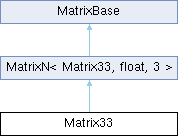MaterialX 1.38.8
Searching...
No Matches
Matrix33 Class Reference

A 3x3 matrix of floating-point values. More...

`#include <Types.h>`

Inheritance diagram for Matrix33:## Public Member Functions

Matrix33 (float m00, float m01, float m02, float m10, float m11, float m12, float m20, float m21, float m22)

Matrix Operations
Matrix33 getTranspose () const
Return the transpose of the matrix.

float getDeterminant () const
Return the determinant of the matrix.

Return the adjugate of the matrix.

Matrix33 getInverse () const
Return the inverse of the matrix.Public Member Functions inherited from MatrixN< Matrix33, float, 3 >
MatrixN (Uninit)

MatrixN (float s)

MatrixN (const float *begin, const float *end)

bool operator== (const Matrix33 &rhs) const
Return true if the given matrix is identical to this one.

bool operator!= (const Matrix33 &rhs) const
Return true if the given matrix differs from this one.

bool isEquivalent (const Matrix33 &rhs, float tolerance) const
Return true if the given matrix is equivalent to this one within a given floating-point tolerance.

RowArray & operator[] (size_t i)
Return the row array at the given index.

const RowArray & operator[] (size_t i) const
Return the const row array at the given index.

Matrix33 operator+ (const Matrix33 &rhs) const

MatrixNoperator+= (const Matrix33 &rhs)

Matrix33 operator- (const Matrix33 &rhs) const
Component-wise subtraction of two matrices.

MatrixNoperator-= (const Matrix33 &rhs)
Component-wise subtraction of two matrices.

Matrix33 operator* (float s) const
Component-wise multiplication of a matrix and a scalar.

MatrixNoperator*= (float s)
Component-wise multiplication of a matrix and a scalar.

Matrix33 operator/ (float s) const
Component-wise division of a matrix by a scalar.

MatrixNoperator/= (float s)
Component-wise division of a matrix by a scalar.

Matrix33 operator* (const Matrix33 &rhs) const
Compute the matrix product.

MatrixNoperator*= (const Matrix33 &rhs)
Compute the matrix product.

Matrix33 operator/ (const Matrix33 &rhs) const
Divide the first matrix by the second (computed as the product of the first matrix and the inverse of the second).

MatrixNoperator/= (const Matrix33 &rhs)
Divide the first matrix by the second (computed as the product of the first matrix and the inverse of the second).

Iterator begin ()

ConstIterator begin () const

Iterator end ()

ConstIterator end () const

float * data ()
Return a pointer to the underlying data array.

const float * data () const
Return a const pointer to the underlying data array.

## Static Public Attributes

static const Matrix33 IDENTITY

## Vector Transformations

Vector3 multiply (const Vector3 &v) const
Return the product of this matrix and a 3D vector.

Vector2 transformPoint (const Vector2 &v) const
Transform the given 2D point.

Vector2 transformVector (const Vector2 &v) const
Transform the given 2D direction vector.

Vector3 transformNormal (const Vector3 &v) const
Transform the given 3D normal vector.

static Matrix33 createTranslation (const Vector2 &v)
Create a translation matrix.

static Matrix33 createScale (const Vector2 &v)
Create a scale matrix.

static Matrix33 createRotation (float angle)
Create a rotation matrix. More...Public Types inherited from MatrixN< Matrix33, float, 3 >
using RowArray = typename std::array< float, N >

using Iterator = typename std::array< RowArray, N >::iterator

using ConstIterator = typename std::array< RowArray, N >::const_iteratorStatic Public Member Functions inherited from MatrixN< Matrix33, float, 3 >
static constexpr size_t numRows ()
Return the number of rows in this matrix.

static constexpr size_t numColumns ()
Return the number of columns in this matrix.Protected Attributes inherited from MatrixN< Matrix33, float, 3 >
std::array< RowArray, N > _arr

## Detailed Description

A 3x3 matrix of floating-point values.

Vector transformation methods follow the row-vector convention, with matrix-vector multiplication computed as v' = vM.

## ◆ createRotation()

 Matrix33 createRotation ( float angle )
static

Create a rotation matrix.

Parameters